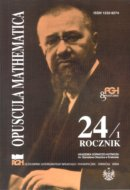Opuscula Math. 24, no. 1 (2004), 85-96

Opuscula Mathematica

# Difference methods for infinite systems of hyperbolic functional differential equations on the Haar pyramid

Danuta Jaruszewska-Walczak

Abstract. We consider the Cauchy problem for infinite system of differential functional equations $\partial_tz_k(t,x)=f_k(t,x,z,\partial_xz_k(t,x)),\;k\in\mathbf{N}.$ In the paper we consider a general class of difference methods for this problem. We prove the convergence of methods under the assumptions that given functions satisfy the nonlinear estimates of the Perron type with respect to functional variables. The proof is based on functional difference inequalities. We constructed the Euler method as an example of difference method.

Keywords: initial problems, infinite systems of differential functional equations, difference functional inequalities, nonlinear estimates of the Perron type.

Mathematics Subject Classification: 65M10, 65M15, 35R10.

Full text (pdf)

• Danuta Jaruszewska-Walczak
• Uniwersytet Gdański, Instytut Matematyki, ul. Wita Stwosza 57, 80-952 Gdańsk, Poland